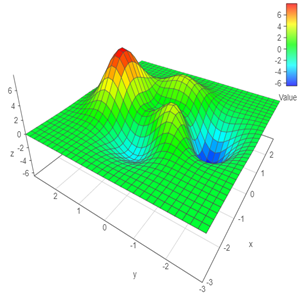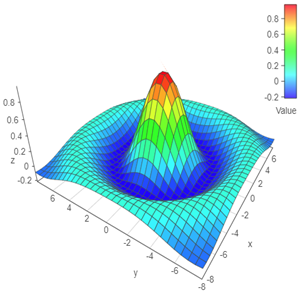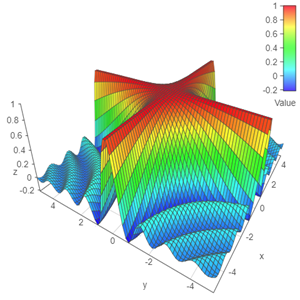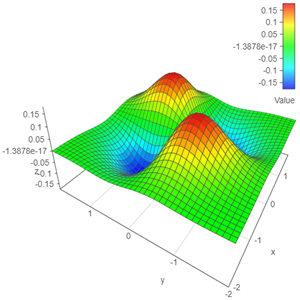### Free Ginckers

#### Simple Chart: Peaks

Template: Graphics/3d-simple-chart

Discription: This template creates a simple 3D chart for a mathematical peaks function with two variables or an x-y-z data set.#### Simple Chart: Sinc

Template: Graphics/3d-simple-chart

Discription: This template creates a simple 3D chart for a mathematical sinc function with two variables or an x-y-z data set.#### Simple Chart: SincXY

Template: Graphics/3d-simple-chart

Discription: This template creates a simple 3D chart for a mathematical sincXY function with two variables or an x-y-z data set.